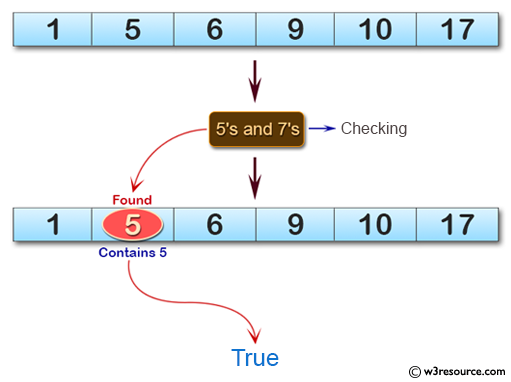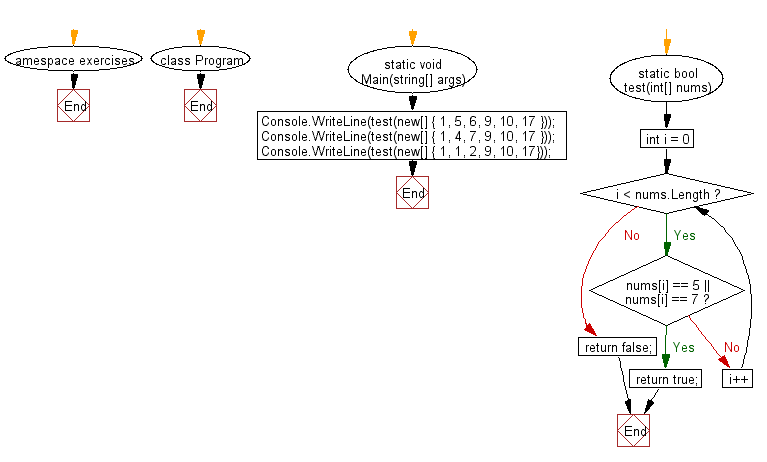﻿ C# - Check whether a array of integers contains 5s or 7s# C# Sharp Basic Algorithm Exercises: Check whether a given array of integers contains 5's or 7's

## C# Sharp Basic Algorithm: Exercise-114 with Solution

Write a C# Sharp program to check whether a given array of integers contains 5's or 7's.

Pictorial Presentation:Sample Solution:

C# Sharp Code:

``````using System;
namespace exercises
{
class Program
{
static void Main(string[] args)
{
Console.WriteLine(test(new[] { 1, 5, 6, 9, 10, 17 }));
Console.WriteLine(test(new[] { 1, 4, 7, 9, 10, 17 }));
Console.WriteLine(test(new[] { 1, 1, 2, 9, 10, 17}));
}
static bool test(int[] nums)
{
for (int i = 0; i < nums.Length; i++)
{
if (nums[i] == 5 || nums[i] == 7) return true;
}

return false;
}
}
}
```
```

Sample Output:

```True
True
False```

Flowchart:C# Sharp Code Editor:

Improve this sample solution and post your code through Disqus

What is the difficulty level of this exercise?

Test your Programming skills with w3resource's quiz.

﻿